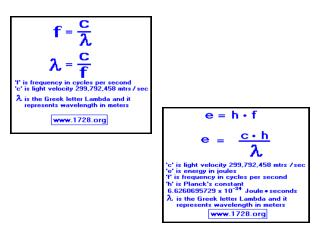DownloadDownload PresentationWavelength, Frequency, and Energy Practice Problems

# Wavelength, Frequency, and Energy Practice Problems

Download Presentation## Wavelength, Frequency, and Energy Practice Problems

- - - - - - - - - - - - - - - - - - - - - - - - - - - E N D - - - - - - - - - - - - - - - - - - - - - - - - - - -
##### Presentation Transcript

1. Wavelength, Frequency, and Energy Practice Problems

2. 1. A certain violet light has a wavelength of 413 nm. What is the frequency of this light?

3. 2. What is the wavelength of radiation whose frequency is 1.50 x 1013 Hz?

4. 3. How much energy is associated with a photon in the microwave region of the spectrum with a frequency of 6.6 x 1012 Hz?

5. 4. A hydrogen lamp emits several lines in the visible region of the spectrum. One of these lines has a wavelength of 6.56 x 10-5 cm. What is the energy of this radiation?

6. 5. A very bright line in the bright-line spectrum of sodium has a wavelength of 590 nm. What is the speed of this wave?